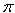SEARCH HOMEMath Central Quandaries & QueriesI've seen the other question about surface areas. I still don't understand the lateral area. In my math book, it has (pi * r * L) as the equation, r = radius and L = height of the cone. This is 8th grade math. Thanks.Hi Michael,

The lateral area of a cone isrL where r is the radius but L is the slant height. Look at what Walter wrote to respond to a similar question a while ago.

PennyMath Central is supported by the University of Regina and The Pacific Institute for the Mathematical Sciences.